Engineering ToolBox - Resources, Tools and Basic Information for Engineering and Design of Technical Applications!

## Online vector calculator - add vectors with different magnitude and direction - like forces, velocities and more.

In mechanics there are two kind of quantities

• scalar quantities with magnitude - time, temperature, mass etc.
• vector quantities with magnitude and direction - velocity, force etc.

When adding vector quantities both magnitude and direction are important. Common methods adding coplanar vectors (vectors acting in the same plane) are

• the parallelogram law
• the triangle rule
• trigonometric calculation

### The Parallelogram Law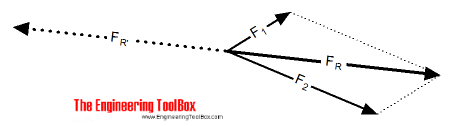The procedure of "the parallelogram of vectors addition method" is

• draw vector 1  using appropriate scale and in the direction of its action
• from the tail of vector 1 draw vector 2 using the same scale in the direction of its action
• complete the parallelogram by using vector 1 and 2 as sides of the parallelogram
• the resulting vector is represented in both magnitude and direction by the diagonal of the parallelogram

### The Triangle Rule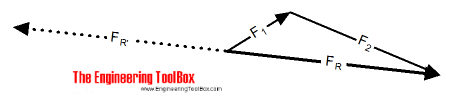The procedure of "the triangle of vectors addition method" is

• draw vector 1 using appropriate scale and in the direction of its action
• from the nose of the vector draw vector 2 using the same scale and in the direction of its action
• the resulting vector is represented in both magnitude and direction by the vector drawn from the tail of vector 1 to the nose of vector 2

### Trigonometric Calculation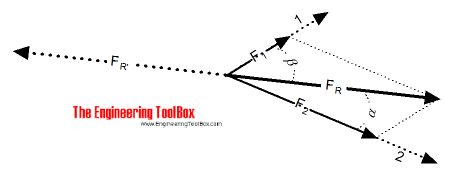The resulting vector of two coplanar vector can be calculated by trigonometry using "the cosine rule" for a non-right-angled triangle.

FR = [F12 + F22 − 2 F1 F2 cos(180o - (α + β))]1/2                          (1)

where

F = the vector quantity - force, velocity etc.

α + β = angle between vector 1 and 2

The angle between the vector and the resulting vector can be calculated using "the sine rule" for a non-right-angled triangle.

α = sin-1[Fsin(180o - (α + β)) / FR]                                     (2)

where

α + β = the angle between vector 1 and 2 is known

A force 1 with magnitude 3 kN is acting in direction 80o from a force 2 with magnitude 8 kN.

The resulting force can be calculated as

FR = [(3 kN)2 + (8 kN)2 - 2 (5 kN) (8 kN) cos(180o - (80o))]1/2

= 9 (kN)

The angle between vector 1 and the resulting vector can be calculated as

α = sin-1[ (3 kN) sin(180o - (80o)) / (9 kN) ]

= 19.1o

The angle between vector 2 and the resulting vector can be calculated as

α = sin-1[ (8 kN) sin(180o - (80o)) / (9 kN) ]

= 60.9o

### Example - Airplane in Wind

A headwind of 100 km/h is acting 30o starboard on an airplane with velocity 900 km/h.

The resulting velocity for the airplane related to the ground can be calculated as

vR = [(900 km/h)2 + (100 km/h)2 - 2 (900 km/h) (100 km/h) cos(180o - (30o))]1/2

= 815 (km/h)

The angle between the airplane course and actual relative ground course can be calculated as

α = sin-1[ (100 km/h) sin((180o) - (30o)) / (815 km/h) ]

= 3.5o

### Vector Calculator

The generic calculator below is based on equation (1) and can be used to add vectors quantities like velocities, forces etc.

### Parallelogram

Resultant vectors can be estimated by drawing parallelograms as indicated below.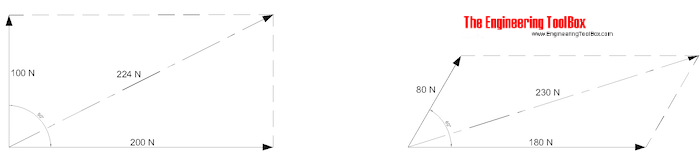1. draw the vectors with right direction and magnitude
2. draw parallel lines to the vectors
3. draw the resultant vector to the crossing point between the parallel lines
4. measure the magnitude and direction of the resultant vector in the drawing

The method can also be used with more than two vectors as indicated below.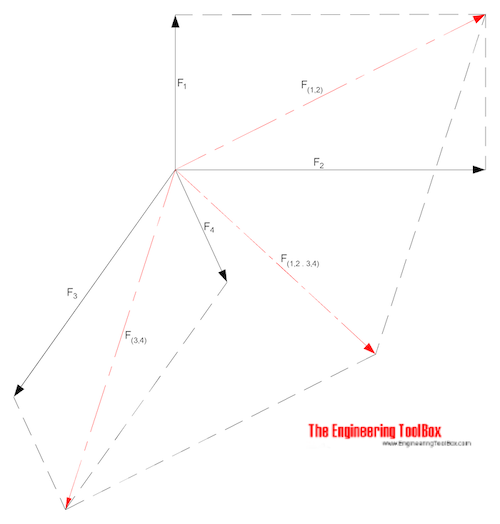1. draw the resultant vectors between two and two vectors
2. draw the resultant vectors between two and two of resultant vectors
3. continue until there is only one final resultant vector
4. measure direction and magnitude of the final resultant vector in the drawing

In the example above - first find the resultant F(1,2) by adding F1 and F2, and the resultant F(3,4) by adding F3 and F4. The find the resultant F(1,2.3,4) by adding F(1,2) and F(3,4).

## Related Topics

• ### Basics

The SI-system, unit converters, physical constants, drawing scales and more.
• ### Dynamics

Motion - velocity and acceleration, forces and torque.
• ### Mathematics

Mathematical rules and laws - numbers, areas, volumes, exponents, trigonometric functions and more.
• ### Mechanics

Forces, acceleration, displacement, vectors, motion, momentum, energy of objects and more.
• ### Statics

Loads - forces and torque, beams and columns.

## Related Documents

• ### Bollard Forces

Friction, load and effort forces acting in ropes turned around bollards.
• ### Complex Numbers

Complex numbers are used in alternating current theory and mechanical vector analysis.
• ### Equilibrant Force

The force required to keep a system of forces in equilibrium.
• ### Force

Newton's third law - force vs. mass and acceleration.
• ### Forces and Tensions in Ropes due to Angle

Reduced load capacities in ropes, cables or lines - due to acting angle.
• ### Law of Cosines

One side of a triangle when the opposite angle and two sides are known.
• ### Oblique Triangle

Calculate oblique triangles.
• ### Pythagorean Theorem

Verifying square corners.
• ### Regular Polygons

Areas of regular polygons - polygons with 3 to 12 sides.
• ### Right Angled Triangle

Right angled triangle equations.
• ### Standard Differentials and Integrals

Equations for differentials and integrals.
• ### Taylor Series

Function as an infinite sum of terms.
• ### Torque or Moment of Force - Online Converter

Torque or moment - the tendency of a force to rotate an object.
• ### Trigonometric Functions

Sine, cosine and tangent - the natural trigonometric functions.

## Engineering ToolBox - SketchUp Extension - Online 3D modeling!

Add standard and customized parametric components - like flange beams, lumbers, piping, stairs and more - to your Sketchup model with the Engineering ToolBox - SketchUp Extension - enabled for use with older versions of the amazing SketchUp Make and the newer "up to date" SketchUp Pro . Add the Engineering ToolBox extension to your SketchUp Make/Pro from the Extension Warehouse !

We don't collect information from our users. More about

## Citation

• The Engineering ToolBox (2008). Vector Addition. [online] Available at: https://www.engineeringtoolbox.com/vector-addition-d_320.html [Accessed Day Month Year].

Modify the access date according your visit.

9.27.11